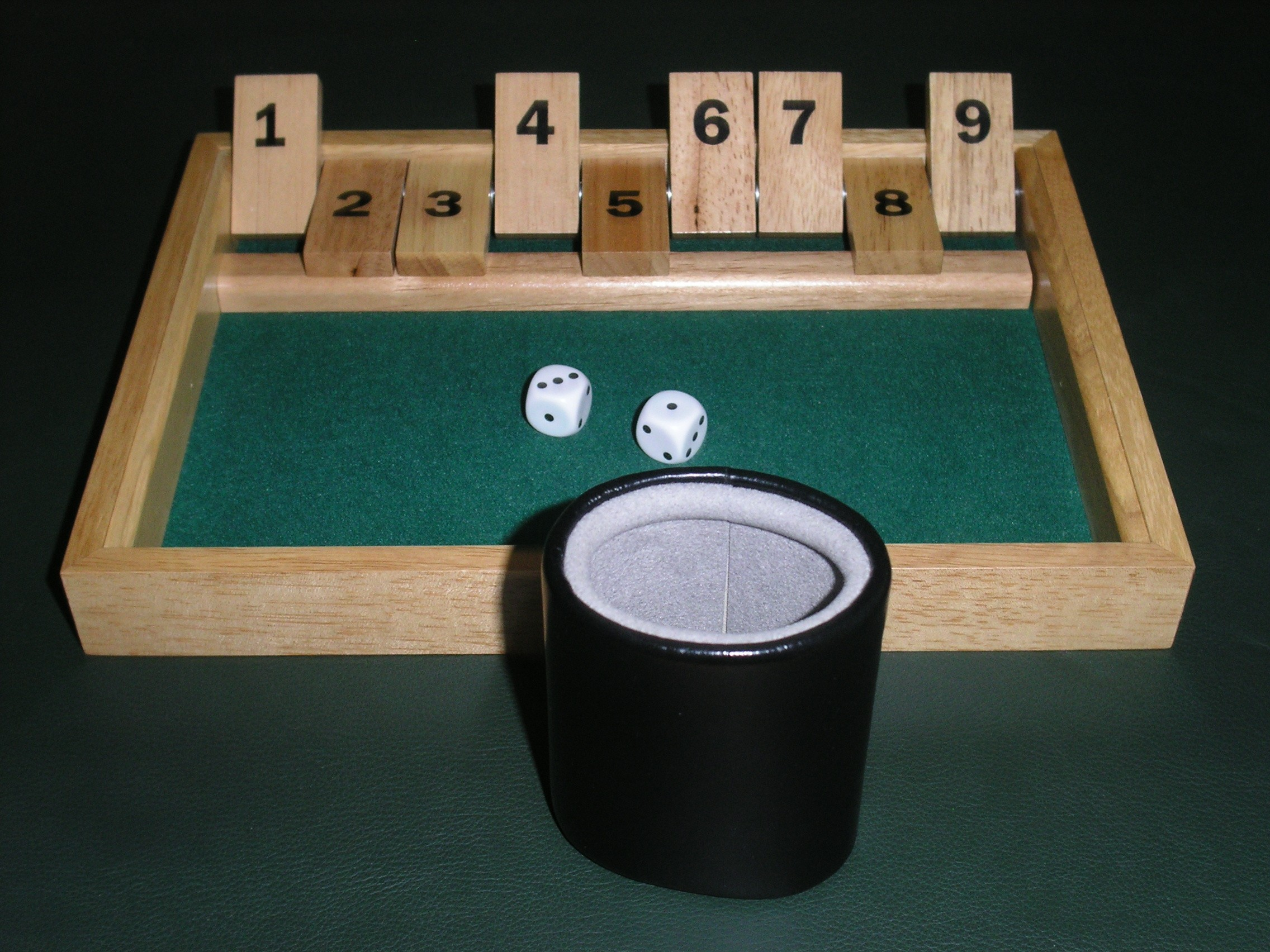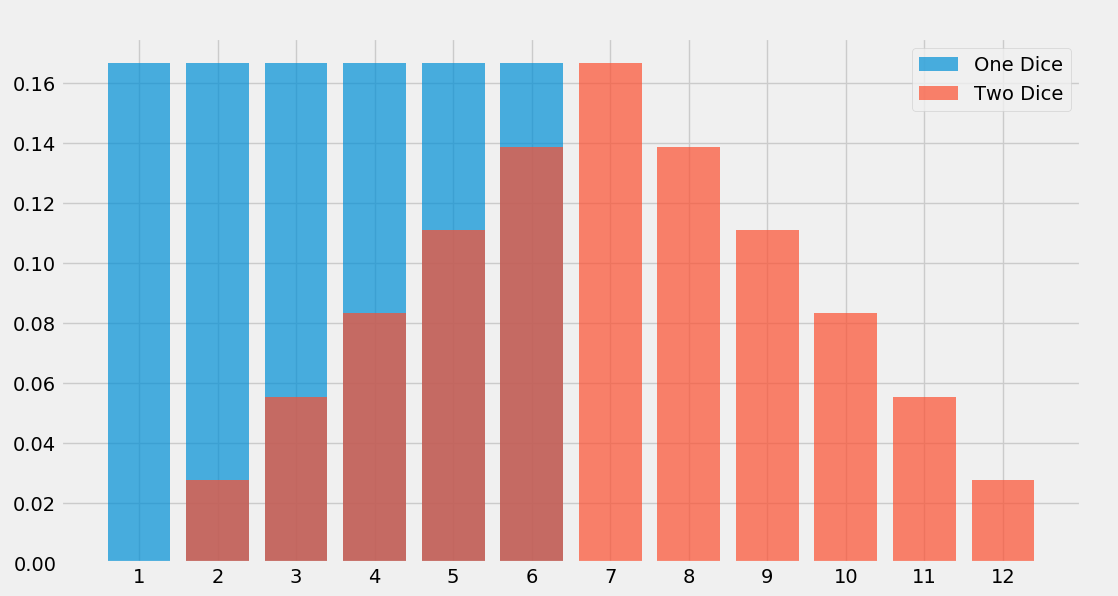A fun game for all the family.

I was in the pub recently and I came across a game I hadn’t seen before. According to Wikipedia it’s got a lot of names, but the name I heard was Shut the Box. It comprises a little wooden box with numbered tiles, a small felt mat, and some dice, like thisThankfully my friend had seen this game before, and knew the rules.1 They’re simple enough, as you can see there are nine numbered tiles, and at the start all tiles are open. The player rolls two dice, and “closes” any combination of open tiles which add up to the numbers shown on the dice, i.e. if you roll a $(2, 3)$ you close $(1, 4)$ or $(2, 3)$ or $(5,)$. You carry on until all the tiles are closed, or until you roll a number that you’ve already closed. There is one small complication, once you’ve closed $7$, $8$, and $9$ you can chose to roll either one or two dice. That’s it.

To give a concrete example of how to play, one straightforward way to win is to roll 4 $(5, 5)$’s, followed by another $(5,)$, like this:

| 1 || 2 || 3 || 4 || 5 || 6 || 7 || 8 || 9 |
dice: 5 5

| _ || 2 || 3 || 4 || 5 || 6 || 7 || 8 || _ |
dice: 5 5

| _ || _ || 3 || 4 || 5 || 6 || 7 || _ || _ |
dice: 5 5

| _ || _ || _ || 4 || 5 || 6 || _ || _ || _ |
dice: 5 5

| _ || _ || _ || _ || 5 || _ || _ || _ || _ |
dice: 5 5

# 7, 8, and 9 are gone. choose to roll 1 dice
| _ || _ || _ || _ || _ || _ || _ || _ || _ |
dice: 5


That was easy. Unfortunately things aren’t always so simple, and you can be unlucky with the dice. For instance if you roll $(1, 1)$ the only thing you can do is close the $2$ (no other combination on the board adds to 2). If you roll another $(1, 1)$ next time round you lose immediately, the $2$ is gone and there aren’t any moves left. Of course this isn’t very likely, it’ll only happen in $1/36 ^ 2 = 0.077\%$ games, but it doesn’t hurt to keep in mind the dice probabilitiesThe obvious question to as here is how likely are you to win a game? Clearly there are some games that are just lost causes, like the pair of $(1,1)$ example above, you can’t possibly win given the dice rolls you’ve gotten. To work out how many are winnable I’ve written a simulator of this simple game. All the code is here. Also, if you clone that repo and run human.py you can play the game yourself.

The core of the code is a function that, given a board state and a dice roll returns all the possible moves – given a number and a list of values return all the sums of values that equal the number. This can be done with a simple recursive function that brute forces all the sums2

def moves(target, numbers=range(1,10), partial=[], partial_sum=0):
if partial_sum == target:
yield partial
if partial_sum >= target:
return
for i, n in enumerate(numbers):
remaining = numbers[i + 1:]
yield from moves(target, remaining, partial + [n], partial_sum + n)


With that function we can simulate a game. What I’m interested in finding out is what percentage of games are theoretically winnable given the dice rolls. To do this I start off with a fully open board and roll the dice. This opens up $n$ possible moves so we create $n$ new boards and make each move. We then loop around, roll the dice again and try all moves on all boards. If a dice roll means that any one of our board results in a win then we call that set of rolls “winnable”, if we end up in a position where the dice roll leaves us with no available moves on any board then that set of rolls is “losing”.

All that’s left to do is play a lot of games, let’s say $10,000$, and see how many we could have (in theory) won.So there we have it, about $70\%$ of games are winnable in theory. Give the game a try next time you’re in the pub, it’s good fun.

1. There seem to be many variants to the rules, I’m not sure that the rules as he taught them to me are canonical but we’ll stick with them.

2. This is a natively quadratic problem, thankfully we’re dealing with small numbers here.